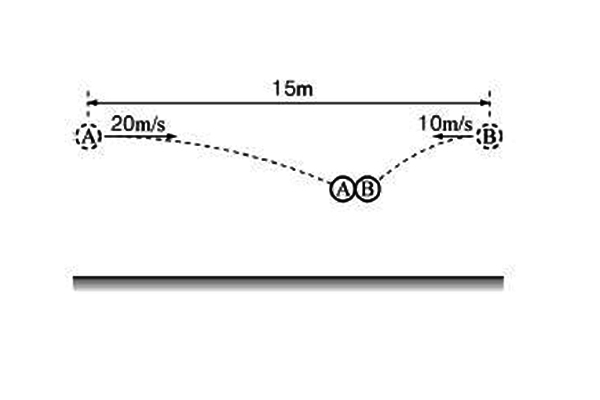# Perfectly inelastic collisionIn the above figure, two objects $A$ and $B$ with the same mass $m$ are $15$ m away from each other. Now, $A$ and $B$ are thrown horizontally at the same time at the velocities $20$ m/s and $-10$ m/s, respectively, eventually colliding with each other in the air. If the two objects stick together after a perfectly inelastic collision, what is the speed of the mass at the moment of collision (in m/s)?

Assume that gravitational acceleration is $g= 10$ m/s$^{2}$.

×